# Rbse Solutions for Class 11 Physics Chapter 10 Mechanical Properties Of Fluids

Rbse Solutions for Class 11 Physics Physics Chapter 10 Mechanical Properties Of Fluids (updated)

NCERT Exercises

Question 1.
Explain why
(a) The blood pressure in humans is greater at the feet than at the brain
(b) Atmospheric pressure at a height of about 6 km decreases to nearly half of its value at the sea level, though the height of the atmosphere is more than 100 km
(c) Hydrostatic pressure is a scalar quantity even though pressure is force divided by area.
(a) The height of the blood column in the human body is more for the feet as compared to that for the brain that is why the blood exerts more pressure at the feet than at the brain.
(b) We know that the density of air is maximum near the surface of earth and decreases rapidly with height and at a height of about 6 km, it decreases to nearly half of its value at the sea level. Beyond 6 km height, the density of air decreases very slowly with height. Due to this reason, the atmospheric pressure at a height of about 6 km decreases to nearly half of its value at sea level.
(c) Since due to the applied force on the liquid, according to Pascal’s law, the pressure is transmitted equally in all directions inside the liquid. Thus there is no fixed direction for the pressure due to liquid. Hence hydrostatic pressure is a scalar quantity.

Question 2.
Explain why
(a) The angle of contact of mercury with glass is obtuse, while that of water with glass is acute.
(b) Water on a clean glass surface tends to spread out while mercury on the same surface tends to form drops. (Put differently, water wets glass while mercury does not.)
(c) Surface tension of a liquid is independent of the area of the surface
(d) Water with detergent dissolved in it should have small angles of contact.
(e) A drop of liquid under no external forces is always spherical in shape.
(a) When a small quantity of liquid is poured on solid, three interfaces namely liquid-air, solid-air and solid- liquid are formed. The surface tensions corresponding to these three interfaces i.e. TLA,TSA and TSL respectively are related to the angle of contact of a liquid with a solid as: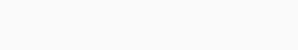In case of mercury-glass, TSA < TSL therefore, from
(i) cos θ is negative or θ > 90° i.e. θ is obtuse. But on the other hand, in case of water- glass, Tsa >TSLi so from (i) cos θ is positive i.e. θ is less than 90° or acute.

(b) For equilibrium of a liquid drop on the surface of a solid, the equation
TSA = TSL + TLA cos θ …(i)
must be satisfied. For mercury-glass, angle of contact is obtuse. In order to achieve this obtuse value of angle of contact, the mercury tends to form a drop.
But in case of water-glass, the angle of contact is acute, so equation (i) is not satisfied. In order to achieve this acute value of angle of contact, the water tends to spread.

(c) Surface tension of liquid is defined as the force acting per unit length on either side of an imaginary line drawn tangentially on the surface of the liquid at rest. Since this force is independent of the area of the liquid surface, therefore surface tension is also independent of the area of the liquid surface.

(d) We know that the cloth has narrow spaces in the form of fine capillaries. The rise of liquid in a capillary tube is given by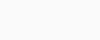i.e. h α cos θ. It follows that if 0 is small, cos θ will be large and detergent will rise more in the narrow spaces in the cloth. Now as detergents having small angles of contact can penetrate more in cloth.

(e) In the absence of external forces, only force acting on the liquid drop is due to surface tension. A drop of liquid tends to acquire minimum surface area due to the property of surface tension. Since for a given volume of liquid, surface area is minimum for a sphere, so the liquid drop will always assume a spherical shape.

Question 3.
Fill in the blanks using the word(s) from the list appended with each statement:
(a)
Surface tension of liquids generally… with temperatures (increases/decreases)
(b) Viscosity of gases … with temperature, whereas viscosity of liquids … with temperature (increases/decreases)
(c) For solids with elastic modulus of rigidity, the shearing force is proportional to …., while for fluids it is proportional to …. (shear strain/rate of shear strain)
(d) For a fluid in a steady flow, the increase in flow speed at a constriction follows…….(conservation of mass/Bernoulli’s principle)
(e) For the model of a plane in a wind tunnel, turbulence occurs at a … speed than for turbulence for an actual plane (greater/ smaller)
(a) decreases
(b) increases; decreases
(c) shear strain; rate of shear strain
(d) conservation of mass of Bernoulli’s principle
(e) greater.

Question 4.
Explain why
(a) To keep a piece of paper horizontal, you should blow over, not under, it.
(b) When we try to close a water tap with our fingers, fast jets of water gush through the openings between our fingers.
(c) The size of the needle of a syringe controls flow rate better than the thumb pressure exerted by a doctor while administering an injection.
(d) A fluid flowing out of a small hole in a vessel results in a backward thrust on the vessel.
(e) A spinning cricket ball in air does not follow a parabolic trajectory
(a) If we blow over a piece of paper, velocity of air above the paper becomes more than that below it. As K.E. of air above the paper increases, so in accordance with
Bernoulli’s theorem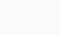2=constant,
its pressure energy and hence its pressure decreases. Due to greater value of pressure below the piece of paper = atmospheric . pressure, it remains horizontal and does not fall.
On the other hand if we blow under the paper, the pressure on the lower side decreases. The atmospheric pressure above the paper will Therefore bend the paper downwards. So the paper will not remain horizontal.
(b) This can be explained from the equation of continuity i.e. = a2υ2. As we try to close a water tap with our fingers, the area of cross-section of the outlet of water jet is reduced considerably as the openings between our fingers provide constriction (i.e., regions of smaller area).
Thus velocity of water increases greatly and fast jets of water come through the openings between our fingers.
(c) According to Bernoulli’s theorem, we know that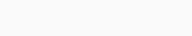…………..(i)
Here, the size of the needle controls the velocity of flow and the thumb pressure controls pressure.
Now P occurs with power one and velocity v occurring with power 2 in equation (i),hence the velocity has more influence. That is why the needle of syringe has a better control over the flow rate.
(d) When a fluid is flowing out of a small hole in a vessel, it acquires a large velocity and hence possesses large momentum. Since no external force is acting on the system, a backward velocity must be attained by the vessel (according to the law of conservation of momentum). As a result of it, backward thrust is experienced by the vessel.
(e) This is due to Magnus effect : Let a ball moving to the right be given a spin at the top of the ball. The velocity of air at the top is higher than the velocity of air below the ball. So according to Bernoulli’s theorem, the pressure above the ball is less than the pressure below the ball. Thus there is a net upward force on the spinning ball, so the ball follows a curved path. This dynamic lift due to spinning is known as Magnus effect.

Question 5.
A 50 kg girl wearing high heel shoes balances on a single heel. The heel is circular with a diameter 1.0 cm. What is the pressure exerted by the heel on the horizontal floor?

Question 6.
Toricelli’s barometer used mercury. Pascal duplicated it using French wine of density 984 kg m-3. Determine the height of the wine column for normal atmospheric pressure.
P= Normal atmospheric pressure = 1.013 x 105 Pa
Let h be the height of the French wine column which earth’s atmosphere can support.
∴ If P’ be the pressure corresponding to height h of wine column,
Then, P’ = hρωg
where ρω = density of wine = 984 kg m -3
Now according to given statement
P’ = P
or hρwg = P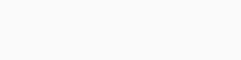Question 7.
A vertical off-shore structure is built to withstand a maximum stress of 109 Pa. Is the structure suitable for putting up on top of an oil well in the ocean? Take the depth of the ocean to be roughly 3 km, and ignore ocean currents.
Here, depth of water column, h = 3 km = 3 x 103 m
density of water, ρ = 103 kg m -3
If P be the pressure exerted by this water column at this depth, then
P = hρg = 3 x 103 x 103 x 9.8
= 29.4 x 106 Pa = 30 x 106 Pa = 3 x 107 Pa
As the structure is put on the sea, sea water will exert upward thrust of 3 x 107 Pa. Maximum stress which the vertical off-shore structure can withstand = 109 Pa. (Given) Then 3 x 107 Pa < 109 Pa Thus we conclude that the structure is suitable as the stress applied by it is much lesser than the maximum stress it can withstand.

Question 8.
A hydraulic automobile lift is designed to lift cars with a maximum mass of 3000 kg. The area of cross-section of the piston carrying the load is 425 cm2. What maximum pressure would the smaller piston have to bear?
The maximum force, which the bigger piston can bear,
F = 3000 kg f = 3000 x 9.8 N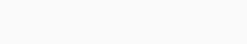Since the liquid transmits pressure equally, therefore the maximum pressure the smaller piston can bear is 6.92 x 105 Pa.

Question 9.
A U-tube contains water and methylated spirit separated by mercury. The mercury columns in the two arms are in level with 10.0 cm of water in one arm and 12.5 cm of spirit in the other. What is the specific gravity of spirit?
Here, for water column in one arm of U-tube :
h1 = 10.0 cm
ρ1 = 1 g cm-3
For spirit column in other arm of U-tube,
h2 = 12.5 cm
p2 = ?Question 10.
In the previous problem, if 15.0 cm of water and spirit each are further poured into the respective arms of the tube, what is the difference in the levels of mercury in the two arms?
(Specific gravity of mercury = 13.6)
On pouring 15 cm of water and spirit each into the respective arms of the U-tube, the mercury level will rise in the arm containing the spirit.
ρw= density of mercury.
Let us select two points A and B lying in the same horizontal plane as shown. Thus according to Pascal’s law,Question 11.

Can Bernoulli’s equation be used to describe the flow of water through a rapid in a river? Explain.
No, Bernoulli’s theorem is used only for stream-line flow.

Question 12.
Does it matter if one uses gauge instead of absolute pressures in applying Bernoulli’s equation? Explain.
No, it does not matter if one uses gauge instead of absolute pressures in applying Bernoulli’s equation, provided the atmospheric pressure at the two points where Bernoulli’s equation is applied are significantly different.

Question 13.
Glycerine flows steadily through a horizontal tube of length 1.5 m and radius 1.0 cm. If the amount of glycerine collected per second at one end is 4.0 x 10-3 kg s-1, what is the pressure difference between the two ends of the tube? (Density of glycerine = 1.3 x 103 kg m3 and viscosity of glycerine = 0.83 Pa s). [You may also like to check if the assumption of laminar flow in the tube is correct].
Here, l = 1.5 m; r = 1 cm = 0.01 m;
q = 0.83 Pa s
mass of the glycerine flowing per sec,
m = 4.0 x 10-3 kg s-1
density of glycerine, p = 1.3 x 103 kg mTherefore, volume of glycerine flowing per second,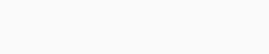Question 14.
In a test experiment on a model aeroplane in a wind tunnel, the flow speeds on the upper and lower surfaces of the wing are 70 m s-1 and 63 m s-1 respectively. What is the lift on the wing if its area is
2.5 m2? Take the density of air to be 1.3 kg m-3.
Let υand υ2 be the speeds on the upper and lower surfaces of the wings of the aeroplane respectively,
P1 and P2 be the pressures on the upper and lower surfaces of the wings respectively.
Here, υ1= 70 m s-1; υ2 = 63 m s-1; p = 1.3 kg m3
The level of the upper and lower surfaces of the wings from the ground may be taken same.
∴ h1-h2
area of wing, A = 2.5 m2
Thus from Bernoulli’s Theorem,Question 15.
Figures (a) and (b) refer to the steady flow of a (non-viscous) liquid. Which of the two figures is incorrect? Why?Figure (a) is incorrect. According to equation of continuity, i.e., aυ= a constant,
where area of cross-section of tube is less, the velocity of liquid flow is more. So the velocity of liquid flow at a constriction of tube is more than the other portion of tube.
According to Bernoulli’s Theorem,2= a constant,
i.e., where υ is more, P is less and vice versa.

Question 16.
The cylindrical tube of a spray pump has a cross-section of 8.0 cm2 on one end of which has 40 fine holes each of diameter 1.0 mm. If the liquid flow inside the tube is 1.5 m min-1, what is the speed of ejection of the liquid through the holes?
Here, cross-section of the tube,
a1 = 8.0 cm2 = 8.0 x 10-4 m2;
The speed of liquid in the tubeQuestion 17.
A U-shaped wire is dipped in a soap solution, and removed. The thin soap film formed between the wire and the light slider supports a weight of 1.5 x 10-2 N (which includes the small weight of the slider). The length of the slider is 30 cm. What is the surface tension of the film?
We know that a soap film has two free surfaces, so total length of the film to be supported, Z = 2 x 30 cm
or l= 60 cm = 0.60 m
Let T = surface tension of the film
If F = total force on the slider due to surface tension, then
P = T x 2l = T x 0.6 N
W= 1.5 x 10-2 N
In equilibrium position, the force F on the slider due to surface tension must be balanced by the weight (W) supported by the slider.Question 18.
Figure (a) shows a thin liquid film supporting a small weight = 4.5 x 10-2 N. What is the weight supported by a film of the same liquid at the same temperature in figure (b) and (c)? Explain your answer physically.(a) Here, length of the film supporting the weight l = 40 cm = 0.4 m
Total weight supported (i.e. force) = 4.5 x 10-2 N. Film has two free surfaces,
∴ Surface tension,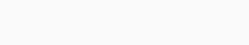Since the liquid is same for all the cases (a), (b) and (c), and temperature is also same, therefore surface tension for cases (b) and (c) will also be the same = 5.625  x 10-2. In figure (b), and (c), the length of the film supporting the weight is also the same as that of (a), hence the total weight supported in each case is 4.5 x 10-2 N.

Question 19.
What is the pressure inside the drop of mercury of radius 3.00 mm at room temperature? Surface tension of mercury at that temperature (20°C) is 4.65 x 10-1 N m1. The atmospheric pressure is 1.01 x 10s Pa. Also give the excess pressure inside the drop.
R = 3.0 mm = 3.0 x 10-3 m;
Surface tension of mercury,
T = 4.65 x 10-1 Nm1
Pressure outside the mercury drop,
P0 = atmospheric pressure = 1.01 x  Pa
If Pi is pressure inside the drop, then excess of pressure inside the mercury drop,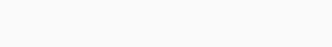Question 20.
What is the excess pressure inside a bubble of soap solution of radius 5.00 mm, given that the surface tension of soap solution at the temperature (20°C) is 2.50 x 10-2 Nm1? If an air bubble of the same dimension were formed at depth of 40.0 cm inside a container containing the soap solution (of relative density 1.20), what would be the pressure inside the bubble? (1 atmospheric pressure is 1.01 x 105 Pa).
Here, surface tension of the soap solution,
T = 2.5 x 102 Nm1
density of the soap solution, p = 1.2 x 103 kg m3;Question 21.
A tank with a square base of area 1.0 m2 is divided by a vertical partition in the middle. The bottom of the partition has a small-hinged door of area 20 cm2. The tank is filled with water in one compartment, and an acid (of relative density 1.7) in the other, both to a height of 4.0 m, compute the force necessary to keep the door closed.
For compartment containing water,
h1 = 4 m, ρ1 = 103 kg m-3.
The pressure exerted by water at the door provided at bottom,To keep the door closed, the force equal to 55 N should be applied horizontally on the door from compartment containing water to that containing acid.

Question 22.
A manometer reads the pressure of a gas in an enclosure as shown in figure, (a). When a pump removes some of the gas, the manometer reads as in figure (b). The liquid used in the manometers is mercury and the atmospheric pressure is 76 cm of mercury.
(a) Give the absolute and gauge pressure of the gas in the enclosure for cases (a) and (b) in units of cm of mercury.
(b) How would the levels change in case (b) if 13.6 cm of water (immiscible with mercury) are poured into the right limb of the manometer? (ignore the small change in the volume of the gas).Here, atmospheric pressure, P = 76 cm of mercury.
(a) In figure (a) pressure head, h = +20 cm
∴ Absolute pressure = P + h = 76 + 20 = 96 cm of mercury
Absolute pressure = P + h = 76 + (-18) = 58 cm of mercury
Gauge pressure = h = -18 cm of mercury
(b) Here 13.6 cm of water added in right limb 13.6 , is equivalent to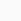= 1 cm of mercury column.
i.e. h’ = 1 cm of mercury column.
Now pressure at A,
Pa = P + h’ = 76 + 1 = 77 cm
Let the difference in mercury levels in the two limbs be hv then pressure at b,
Pb = 58 + h1,
As, Pa = Pb’
∴ 77 = 58 + h1, or h1 = 77 – 58 = 19 cm of mercury column.

Question 23.
Two vessels have the same base area but different shapes. The first vessel takes twice the volume of water that the second vessel requires to fill upto a particular common height. Is the force exerted by the water on the base of the vessels the same in the two cases? If so, why do the vessel filled with water to that same height give different readings on a weighing scale?
Since the pressure depends upon the height of water column and the height of the water column in the two vessels of different shapes is the same, hence there will be same pressure due to water on the base of each vessel. As the base area of each vessel is same, hence there will be equal force acting on the two base areas due to water pressure. The water exerts force on the walls of the vessel also. In case, the walls of the vessel are not perpendicular to base, the force exerted by water on the walls has a net non-zero vertical component which is more in first vessel than that of second vessel. That is why, the two vessels filled with water to same vertical height show different readings on a weighing machine.

Question 24.
During blood transfusion the needle is inserted in a vein where the gauge pressure is 2000 Pa. At what height must the blood container be placed so that blood may just enter the vein?
(Density of blood = 1.06 x 103 kg m-3)
Here, gauge pressure, P = 2000 Pa
density of whole blood, ρ = 1.06 x 103 kg m3
g = 9.8m s-2
Let h = height at which blood container must be placed = ?
Using the relation, P = hρg, we getQuestion 25.
In deriving Bernoulli’s equation, we equated the work done on the fluid in the tube to its change in the potential and kinetic energy.
(a) How does the pressure changes as the fluid moves along the tube if dissipative forces are present? (b) Do the dissipative forces become more important as the fluid velocity increases? Discuss qualitatively.
(a) While deriving Bernoulli’s equation, we say that, decrease in pressure energy per second = increase in K.E./sec. + increase in P.E./sec. Assume that viscous forces are absent. Thus as the fluid flows from lower to upper edge, there is a fall of pressure energy due to the fall of pressure. If dissipating forces are present, then a part of this pressure energy will be used in overcoming these forces during the flow of fluid. So there shall be greater drop of pressure as the fluid moves along the tube.
(b) Yes, the dissipative forces become more important as the fluid velocity increases. According to Newton’s law of viscous drag,
we know that F = -ηA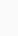Clearly as v increases, velocity gradient increases and hence viscous drag i.e. dissipative force also increases.

Question 26.
(a) What is the largest average velocity of blood flow in an artery of radius 2 x 10-3 m if the flow must remain laminar?
(b) What is the corresponding flow rate? (Take viscosity of blood to be 2.084 x 10-3 Pa s). Density of blood is 1.06 x 103 kg/m3
Here, p = 2 x 10-3 m;
D = 2r = 2 x 2 x 10-3 = 4 x 10-3 m;
η= 2.084 x 10-3 Pa s,
For flow to be laminar, NR = 2000Question 27.
A plane is in level flight at constant speed and each of its two wings has an area of 25 m2. If the speed of the air is 180 km h-1 over the lower wing and 234 km h-1 over the upper wing surface, determine the plane’s mass. (Take air density to be 1 kg m3).
Here, υ1 = 180 km h-1 = 50 m s-1, υ2 = 234 km h-1 = 65 m s-1
area of the each wing, A = 25 m2
and density of air, ρ= 1 kg m 3
According to Bernoulli’s theorem,Question 28.
In Millikan’s oil drop experiment, what is the terminal speed of an uncharged drop of radius
2.0 x 10 5 m and density 1.2 x 103 kg m3? Take the viscosity of air at the temperature of the experiment to be 1.8×10-5 Pa s. How much is the viscous force on the drop at that speed? Neglect buoyancy of the drop due to air.
Here, r = 2x 10-5m, q = 1.8 x 10-5 Pa s,
p = 1.2 x 103 kg m-3
When the buoyancy of the drop due to air is neglected, the terminal speed of the drop is given byQuestion 29.
Mercury has an angle of contact equal to 140° with soda lime glass. A narrow tube of radius mm made of this glass is dipped in a trough containing mercury. By what amount does the mercury dip down in the tube relative to the liquid surface outside? Surface tension of mercury at the temperature of the experiment is 0.465 N m-1. Density of mercury = 13.6 x 103 kg m-3.
Here, angle of contact, θ = 140°
r = 1 mm = 10-3 m
Surface tension, T = 0.465 N m_1
density of mercury, ρ = 13.6 x 103 kg m-3

= – 5.34 x 10-3 m = – 5.34 mm.
Here negative sign shows that the mercury level is depressed in the tube relative to the mercury surface outside

Question 30.
Two narrow bores of diameters 3.0 mm and 6.0 mm are joined together to form a U-tube open at both ends. If the U-tube contains water, what is the difference in its levels in the two limbs of the tube? Surface tension of water at the temperature of the experiment is 7.3 x 10-2N m-1. Take the angle of contact to be zero and density of water to be 10 x 103 kg m-3 (g = 9.8 m s-2).
Here, T = 7.3 x 10-2 Nm-1
ρ = 1.0 x 103 kg m-3; θ = 0°
For narrow tube, 2r1 = 3.00 mm = 3 x 10-3 m or r1 = 1.5 x 10-3 m
For wider tube, 2r2 = 6.00 mm = 6 x 10-3 m or r2 = 3 x 10-3 m
Let h1 h2 be the heights to which water rises in narrow tube and wider tube respectivelyQuestion 31.
(a) It is known that density r of air decreases with height r as………….
whereρ0 = 1.25 kg m-3 is the density at sea level, and y0 is a constant. This density variation is called the law of atmospheres. Obtain this law assuming that the temperature of atmosphere remains a constant (isothermal conditions). Also assume that the value of g remains constant.
(b) A large He balloon of volume 1425 m3 is used to lift a payload of 400 kg. Assume that the balloon maintains constant radius as it rises. How high does it rise?
[Take y0 = 8000 m and ρ0He  = 0.18 kg m3]